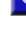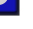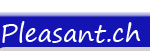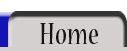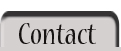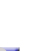All Statements Dim Statement ReDim StatementSTATEMENT:  Erase Implemented in version 1.0  Erase   The Erase statement is used to empty arrays.   If the array is fixed (static), this statement removes the values for all of the elements. If the fixed array is a string array, all of the string elements are reinitialized to "". If the fixed array is a numeric array, all of the numeric elements are reinitialized to 0. So the memory remains allocated for the elements. The array continues to exist with the same size (in the example 3), but the elements are essentially zeroed out.   Fixed array:   Code: <% Dim myarray(3) %> <% myarray(0) = 111 %> <% myarray(1) = 222 %> <% myarray(2) = 333 %> <% Erase myarray %>   If the array is dynamic, the erase statement frees the memory allocated to the dynamic array and the array is destroyed. If you try to access an element in the erased array, you will get an error message (array out of bounds) since the elements do not exist in memory any more. However, you can reinitialize the array by using ReDim. This ability to free memory is very useful.   Dynamic array:   Code: <% Dim anarray() %> <% ReDim anarray(3) %> <% anarray(0) = "First string." %> <% anarray(1) = "Second string." %> <% anarray(2) = "Third string." %> <% Erase anarray %>